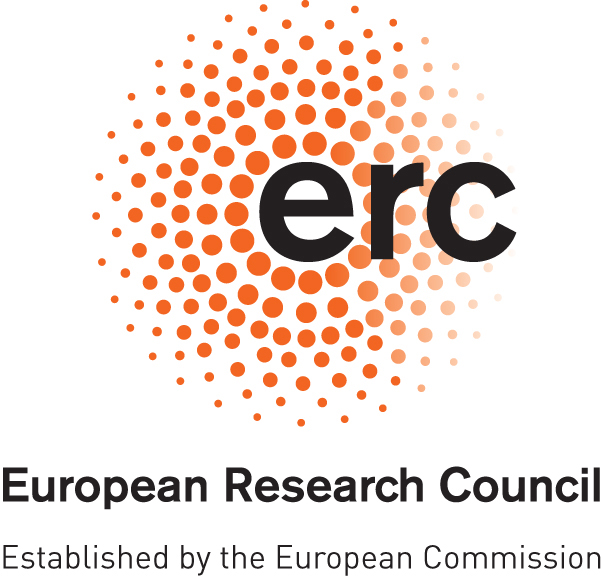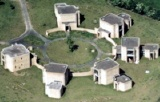# One Day Birmingham-Warwick Combinatorics Meeting

#### 9th June 2017

The Universities of Birmingham and Warwick both have large and active research groups in Combinatorics, and many common research interests. Being so close in location, it makes sense to have a joint meeting. On 9th June 2017 we will gather at the University of Warwick to hear several speakers give talks on combinatorial topics (this will replace the usual Combinatorics seminar). The event will take place in the Zeeman building and all talks will be held in MS.05. All are welcome to attend and no registration is required but please email K dot L dot Staden at warwick dot ac dot uk if you plan on attending.

Organisers: Oleg Pikhurko (O dot Pikhurko at warwick dot ac dot uk) and Katherine Staden (K dot L dot Staden at warwick dot ac dot uk)

###### Schedule
• 14:50--15:40 Yury Person (Frankfurt)
• 15:40--16:00 Coffee
###### Titles and Abstracts

10:30--11:20 Andrzej Ruciński

Title: $\frac{5}{9}$ or Minimum Vertex Degree Condition for Tight Hamiltonian Cycles in 3-Uniform Hypergraphs

Abstract: The study of Dirac type questions for hypergraphs was initiated by G.Y. Katona and H. Kierstead in 1999. Improving upon earlier results, we show that every 3-uniform hypergraph with $n$ vertices and minimum degree at least $(5/9+o(1))\binom{n-1}2$ contains a tight Hamiltonian cycle. Owing to known lower bound constructions, this degree condition is asymptotically optimal. This is joint work with Ch. Reiher, V. Rödl, M. Schacht, and E. Szemerédi.

11:20--12:10 John Haslegrave

Title: The speed of reaching consensus on a graph

Abstract: We consider a random model of opinion-forming on graphs, where some opinions may be more persuasive than others. In the case of regular graphs this process is a generalisation of the voter model, and we show that the complete graph achieves consensus fastest among regular graphs, answering a conjecture of Donnelly and Welsh. The case where one opinion is completely dominant may be thought of as modelling information spread, and we give an asymptotically tight upper bound on the time taken in this case. We also give a monotonicity result on biased random walks with symmetric delays, which applies in the case of the complete graph. Our results raise many open questions. This is joint work with Mate Puljiz (Birmingham).

14:00--14:50 Jaehoon Kim

Title: Sparse $k$-connected subgraphs in tournaments

Abstract: In 2009, Bang-Jensen asked whether there exists a function $g(k)$ such that every strongly $k$-connected $n$-vertex tournament contains a strongly $k$-connected spanning subgraph with at most $kn + g(k)$ arcs. In this talk, we answer the question by showing that every strongly $k$-connected $n$-vertex tournament contains a strongly $k$-connected spanning subgraph with at most $kn + 750k^2\log_2(k+1)$ arcs. This is joint work with Dong Yeap Kang, Younjin Kim and Seewon Suh.

14:50--15:40 Yury Person

Title: Symmetric and asymmetric Ramsey properties in random hypergraphs

Abstract: A celebrated result of Rödl and Ruciński states that for every graph $$F$$, which is not a forest of stars and paths of length $3$, and fixed number of colours $$r\ge 2$$ there exist positive constants $$c, C$$ such that for $$p \leq cn^{-1/m_2(F)}$$ the probability that every colouring of the edges of the random graph $$G(n,p)$$ contains a monochromatic copy of $$F$$ is $$o(1)$$ (the ''0-statement''), while for $$p \geq Cn^{-1/m_2(F)}$$ it is $$1-o(1)$$ (the ''1-statement''). Here $m_2(F)$ denotes the $2$-density of $F$. On the other hand, the case where $F$ is a forest of stars has a coarse threshold which is determined by the appearance of a certain small subgraph in $G(n, p)$.

Recently, the natural extension of the 1-statement of this theorem to $$k$$-uniform hypergraphs was proved by Conlon and Gowers and, independently, by Friedgut, Rödl and Schacht. In particular, they showed an upper bound of order $n^{-1/m_k(F)}$ for the $1$-statement, where $m_k(F)$ denotes the $k$-density of $F$. Similarly as in the graph case, it is known that the threshold for star-like hypergraphs is given by the appearance of small subgraphs. We show that another type of thresholds exists if $k \ge 4$: there are $k$-uniform hypergraphs for which the threshold is determined by the asymmetric Ramsey problem in which a different hypergraph has to be avoided in
each colour-class.

We also obtain a general bound on the $1$-statement for asymmetric Ramsey properties in random hypergraphs. This extends the work of Kohayakawa and Kreuter, and of Kohayakawa, Schacht and Spöhel who showed a similar result in the graph case. We prove the corresponding 0-statement for hypergraphs satisfying certain balancedness conditions.

This is joint work with Gugelmann, Nenadov, Škorić, Steger and Thomas.

###### Travel tips from University station / Birmingham New Street

There are two main options for travelling to the Warwick campus from University station via public transport:

• Train to Coventry station (around 45 minutes, ~£6 for an off-peak return), then bus 11, 11x, or 12x to the Interchange on Warwick campus (£4 for a day ticket). The 12x is direct. The Zeeman building is then a 5 minute walk from here.
• Train to Canley (around 45 minutes, ~£6 for an off-peak return), then walk 20-30 minutes to the Zeeman building.

This conference is supported by Starting Grant No. 306493 of the European Research Council (ERC).Internet Access at Warwick:
Where possible, visitors should obtain an EDUROAM account from their own university to enable internet access whilst at Warwick.
If you need WiFi whilst at Warwick, click here for instructions (upon arrival at Warwick)
Registration:
You can register for any of the symposia or workshops online. To see which registrations are currently open and to submit a registration, please click hereLink opens in a new window.
Contact:
Mathematics Research Centre
Zeeman Building
University of Warwick
Coventry CV4 7AL - UK
E-mail:
MRC@warwick.ac.uk